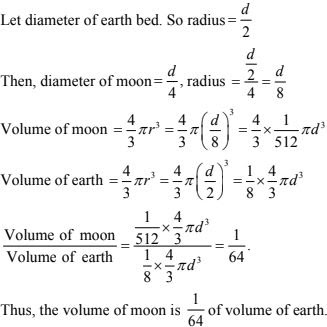# Chapter 21 Surface Area and Volumes of a Sphere RD Sharma Solutions Exercise 21.2 Class 9 Maths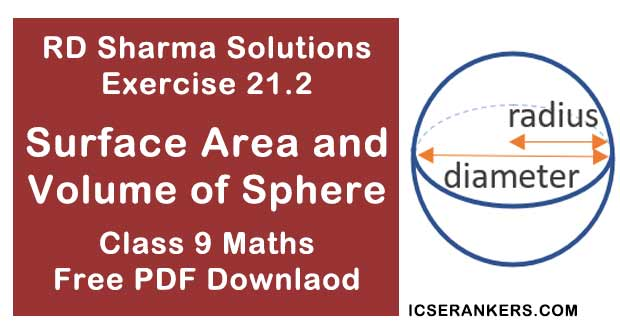Chapter Name RD Sharma Chapter 21 Surface Area and Volumes of a Sphere Exercise 21.2 Book Name RD Sharma Mathematics for Class 10 Other Exercises Exercise 21.1 Related Study NCERT Solutions for Class 10 Maths

### Exercise 21.2 Solutions

1. Find the volume of a sphere whose radius is:
(i) 2 cm

(ii) 3.5 cm

(iii) 10.5 cm

Solution

(i) Radius (r) = 2 cm
∴ Volume = 4/3πr3
= 4/3 × 22/7 × (2)3 = 33.52 cm3

(ii) Radius (r) = 3.5 cm
∴ Volume = (3.5)3 × π × 4/3
= 4/3 × 22/7 × (3.5)3
= 179.666 cm3

(iii) Radius (r) = 10.5 cm
Volume = 4/3πr3
= 4/3 × 22/7 × (10.5)3
= 4851 cm3

2. Find the volume of a sphere whose diameter is :
(i) 14 cm

(ii) 3.5 dm

(iii) 2.1 m

Solution

(i) Diameter = 14 cm, radius = 14/2 = 7 cm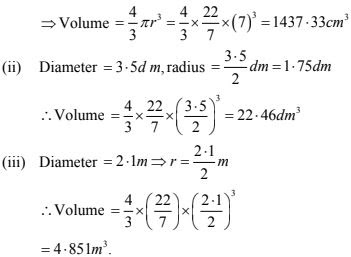3. A hemispherical tank has inner radius of 2.8 m. Find its capacity in litres.

Solution

Radius of tank = 2.8 m
∴ Capacity = 2/3 × 22/7 × (2.8)3 = 45.994 m3
∴ Capacity in liters = 45994 liters [1m3 = 1000]

4. A hemispherical bowl is made of steel 0.25 cm thick. The inside radius of the bowl is 5 cm. Find the volume of steel used in making the bowl.

Solution

Outer radius = 5 + 0.25 = 5.25
Volume of steel used = outer volume - inner volume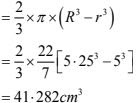5. How many bullets can be made out of a cube of lead, whose edge measures 22 cm, each bullet being 2 cm in diameter ?

Solution

Cube edge = 22 cm
∴ Volume of cube = (22)3   = 10648 cm3
And,
Volume of each bullet  = 4/3 πr3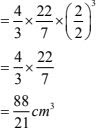∴ No. of bullets = Volume of cube/Volume of bullet
= 10648/88/21 = 2541

Solution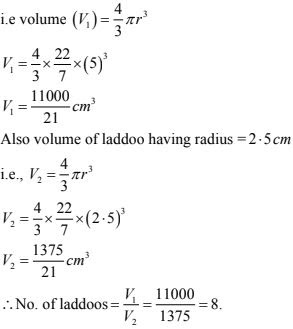7. A spherical ball of lead 3 cm in diameter is melted and recast into three spherical balls. If the
diameters of two balls be 3/2 cm and 2 cm, find the diameter of the third ball.
Solution
Volume of lead ball = 4/3 πr3
= 4/3 × 22/7 × (3/2)3
∴ According to question,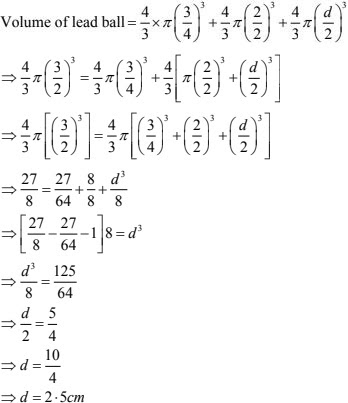8. A sphere of radius 5 cm is immersed in water filled in a cylinder, the level of water rises 5/3 cm. Find the radius of the cylinder.
Solution
Volume of sphere = 4/3 πr3 = 4/3π(5)3
∴ Volume of water rise in cylinder = Volume of sphere
Let r be the radius of the cylinder9. If the radius of a sphere is doubled, what is the ratio of the volume of the first sphere to that of the second sphere?
Solution
Let V1 and V2 be the volumes of first sphere and second sphere respectively
Radius of 1st sphere = r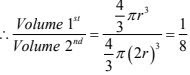10. A cone and a hemisphere have equal bases and equal volumes. Find the ratio of their heights.
Solution
Given that
Volume of thee cone = Volume of the hemisphere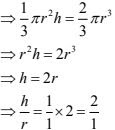∴ Ratio of the their height is 2 : 1.

11. A vessel in the form of a hemispherical bowl is full of water. Its contents are emptied in a right circular cylinder. The internal radii of the bowl and the cylinder are 3.5 cm and 7 cm respectively. Find the height to which the water will rise in the cylinder.
Solution
Given that
Volume of water in the hemisphere bowl = Volume of water in the cylinder
Let n be the height to which water rises in the cylinder.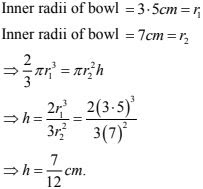12. A cylinder whose height is two thirds of its diameter, has the same volume as a sphere of radius 4 cm. Calculate the radius of the base of the cylinder.
Solution
Given that,
Height of cylinder = 2/3 (diameter)
We know that,
h = 2/3 × 2r = 4/3 r
Volume of the cylinder = volume of the sphere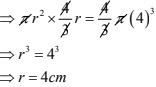13. A vessel in the form of a hemispherical bowl is full of water. The contents are emptied into a cylinder. The internal radii of the bowl and cylinder are respectively 6 cm and 4 cm. Find the height of water in the cylinder.
Solution
It is given that,
Volume of water is hemisphere bowl = Volume of cylinder
⇒ 2/3 π(6)3 = π(4)2 h
⇒ h = 2/3 × (6×6×6)/(4×4)

14. A cylindrical tub of radius 16 cm contains water to a depth of 30 cm. A spherical iron ball is dropped into the tub and thus level of water is raised by 9 cm. What is the radius of the ball ?
Solution
Let r be the radius of the iron ball
Then, Volume of iron ball  = Volume of water raised in the hub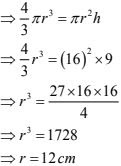Therefore, radius of the ball = 12 cm.

15. A cylinder of radius 12 cm contains water to a depth of 20 cm. A spherical iron ball is dropped into the cylinder and thus the level of water is raised by 6.75 cm. Find the radius of the ball. (Use π = 22/7)
Solution
Given that, Radius of cylinder = 12 cm = r1
Raised in height = 6.75 cm = h
⇒ Volume of water raised = Volume of the sphere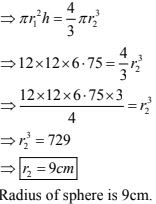16. The diameter of a coper sphere is 18 cm. The sphere is melted and is drawn into a long wire of uniform circular cross-section. If the length of the wire is 108 m, find its diameter.
Solution
Given that diameter of a coper sphere = 18 cm.
Radius of the sphere = 9 cm
Length of the wire = 108 m = 10800 cm
Volume of cylinder = volume of sphere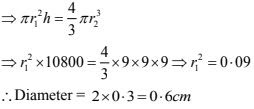17. A cylindrical jar of radius 6 cm contains oil. Iron spheres each of radius 1 .5 cm are immersed in the oil. How many spheres are necessary to raise the level of the oil by two centimetres?
Solution
Given that,
Radius of cylinder jar = 6 cm = r1
Level to be rised = 2cm = h
Radius of each iron sphere = 1.5 cm  =r2
Number of sphere = Volume of cylinder/Volume of sphere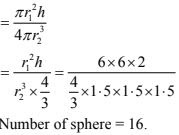18. A measuring jar of internal diameter 10 cm is partially filled with water. Four equal spherical balls of diameter 2 cm each are dropped in it and they sink down in water completely. What will be the change in the level of water in the jar?
Solution
Given that,
Diameter of jar = 10cm
Let the level of water raised by ‘h’
Diameter of spherical ball = 2cm
Volume of jar = 4(Volume of spherical)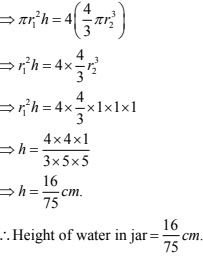19. The diameter of a sphere is 6 cm. It is melted and drawn into a wire of diameter 0.2 cm. Find the length of the wire.
Solution
Given, that,
Diameter of sphere = 6 cm
Radius of sphere = d/2 = 6/2 cm = 3 cm = r1
Diameter of the wire = 0.2 cm
Radius of the wire = 0.1 cm  =r2
Volume of sphere = Volume of wire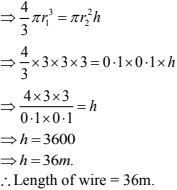20. The radius of the internal and external surfaces of a hollow spherical shell are 3 cm and 5 cm  respectively. If it is melted and recast into a solid cylinder of height 8/3 cm. Find the diameter of the cylinder.
Solution
Given that,
Internal radius of the sphere = 3 cm  = r1
External radius of the sphere = 5 cm  = r2
Height of cylinder = 8/3 m = h
Volume of spherical shell = Volume of the cylinder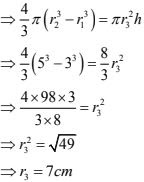∴ Diameter of the cylinder = 2 (radius) = 14 cm

21. A hemisphere of lead of radius 7 cm is cast into a right circular cone of height 46 cm. Find the radius of the base.
Solution
Given radius of hemisphere = 7 cm = r1
Height of cone h = 49 cm
Volume of hemisphere = Volume of cone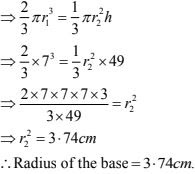22. A hollow sphere of internal and external radii 2 cm and 4 cm respectively is melted into a cone of base radius 4 cm. Find the height and slant height of the cone.
Solution
Given that
Hollow sphere external radii = 4cm = r2
Internal radii (r1) = 2 cm
Cone base radius (R) = 4 cm
Height = ?
Volume of cone = Volume of sphere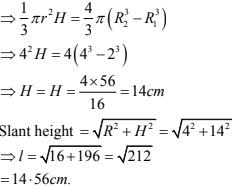23. A metallic sphere of radius 10.5 cm is melted and thus recast into small cones, each or radius 3.5 cm and height 3 cm. Find how many cones are obtained.
Solution
Given that,
Metallic sphere of radius  = 10.5 cm
Height of radius = 3 cm
Let the number of cones obtained be x.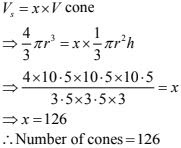24. A cone and a hemisphere have equal bases and equal volumes. Find the ratio of their heights.
Solution
Given that
A cone and a hemisphere have equal bases and equal volumes. Find the ratio of their heights.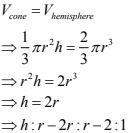25. A cone, a hemisphere and a cylinder stand on equal bases and have the same height. Show that their volumes are in the ratio 1 : 2: 3.
Solution
Given that,
A cone, hemisphere and a cylinder stand one equal bases and have the same weight
We know that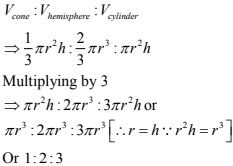26. A cylindrical tub of radius 12 cm contains water to a depth of 20 cm. A spherical form ball
is dropped into the tub and thus the level of water is raised by 6.75 cm. What is the radius of
the ball?
Solution
A cylindrical tub of radius = 12 cm
Depth = 20 cm.
Let r cm be the radius of the ball
Then, volume of ball = Volume of water raised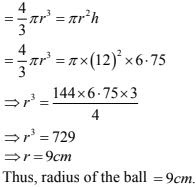27. The largest sphere is carved out of a cube of side 10.5 cm. Find the volume of the sphere.
Solution
Given that,
The largest sphere is carved out of a cube of side = 10.5 cm
Volume of the sphere = ?
We have,
Diameter of the largest sphere = 10.5 cm
2r = 10.5
⇒ r = 5.25 cm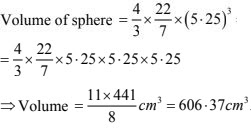28. A sphere, a cylinder and a cone have the same diameter. The height of the cylinder and also
the cone are equal to the diameter of the sphere. Find the ratio of their volumes.
Solution
Let r be the common radius thus,
h = height of the cone = Height of the cylinder = 2r
Let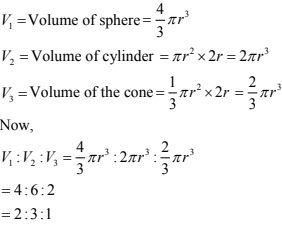29. A cube of side 4 cm contains a sphere touching its side . Find the volume of the gap in between.
Solution
It is given that
Cube side = 4 cm
Volume of cube = (4 cm)3 = 64 cm3
Diameter of the sphere = Length of the side of the cube = 4 cm
∴ Radius of sphere = 2 cm
Volume of the sphere = 4/3 πr3
= 4/3 × 22/7 × (2)3 = 33.52 cm3
∴ Volume of gap = Volume of cub - Volume of sphere
= 64 cm3 - 33.52 cm3 = 30.48 cm3

30. A hemispherical tank is made up of an iron sheet 1 cm thick. If the inner radius is 1 m, then
find the volume of the iron used to make the tank.
Solution
Given that,
Inner radius (r1 ) of hemispherical tank = 1m = r1
Thickness of hemispherical tank = 1 cm  = 0.01m
Outer radius (r2 ) of the hemispherical = (1 + 0.01 m) = 1.01 m = r2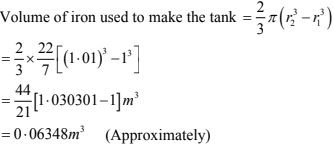31. A capsule of medicine is in the shape of a sphere of diameter 3.5 mm. How much medicine (in mm3) is needed to fill this capsule ?
Solution
Given that,
Diameter of capsule = 3.5mm
Radius = 3.5/2 = 1.75 mm
Volume of spherical capsule = 4/3 πr3
= 4/3 × 22/7 × (1.75)3 mm3
= 22.458 mm3
∴ 2.46 mm3 of medicine is required.

32. The diameter of the moon is approximately one fourth of the diameter of the earth. What
fraction of the volume of the earth is the volume of the moon?
Solution
Given that,
The diameter of the moon is approximately one fourth of the diameter of the earth.